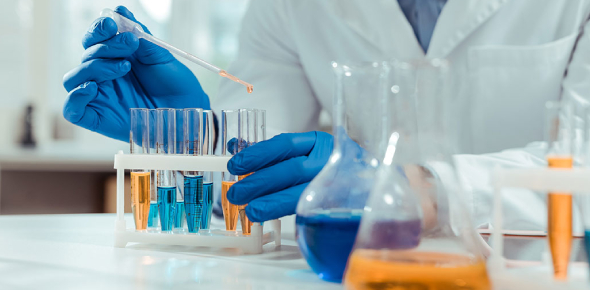# Basics Of Chemistry Exam: Quiz!

50 Questions | Total Attempts: 58SettingsDo you like chemistry? Chemistry is the systematic discipline concerned with elements and compounds that are made up of matter. It studies their composition, structure properties, behavior, and transformations during exposure to other substances. It explores the way that these substances react, unite and change. They utilize unique processes to facilitate the conception of new materials. This quiz is for anyone who is learning about chemistry. This quiz can be helpful. Go for it.

• 1.
The rapid oxidation of a substance, accompanied by the production of heat and light is called?
• A.

Combustion

• B.

Reduction

• C.

Emulsification

• D.

Ionization

• 2.
Which of the following is NOT a physical mixture?
• A.

Element

• B.

Solution

• C.

Suspension

• D.

Emulsion

• 3.
Two or more immiscible substances combine with a special binding ingredient to create a(n)?
• A.

Emulsion

• B.

Solution

• C.

Suspension

• D.

Solvent

• 4.
When acids & alkalis are mixed together in equal proportions, they form?
• A.

Water

• B.

Salt

• C.

Carbon

• D.

Nitrogen

• 5.
Any substance that occupies space & has mass (weight) is considered?
• A.

Matter

• B.

Light

• C.

Energy

• D.

Carbon

• 6.
Which of the following is an example of a chemical property?
• A.

Glossiness

• B.

Solubility

• C.

Melting point

• D.

Ability of wood to burn

• 7.
If two liquids are mutually soluble, they are considered?
• A.

Miscible

• B.

Immiscible

• C.

Suspensions

• D.

Pure

• 8.
A(n) _________ releases a significant amount of heat?
• A.

Exothermic reaction

• B.

Chemical change

• C.

Physical change

• D.

Endothermic reaction

• 9.
A(n) ________ is an alkaline substance used to neutralize acids or raise the pH of hair products?
• A.

Volatile alcohol

• B.

Glycerin

• C.

Alkanolamine

• D.

Silicone

• 10.
Characteristics of matter that do NOT involve chemically changing a substance are called __________ properties.
• A.

Static

• B.

Primary

• C.

Physical

• D.

Chemical

• 11.
Volatile organic compounds contain ________ & evaporate very easily.
• A.

Carbon

• B.

Nitrogen

• C.

Oxygen

• D.

Hydrogen

• 12.
When stored under ideal conditions, a properly formulated emulsion can be stable for up to?
• A.

6 months

• B.

1 year

• C.

3 years

• D.

3 months

• 13.
Matter does NOT exist in which form?
• A.

Solid

• B.

Liquid

• C.

Gas

• D.

Energy

• 14.
A chemical combination of two or more atoms of different elements in definite proportions is a(n)?
• A.

Elemental molecule

• B.

Ion

• C.

Element

• D.

Compound

• 15.
The term pH represents the quantity of _______ ions.
• A.

Nitrogen

• B.

Hydrogen

• C.

Carbon

• D.

Oxygen

• 16.
Pure water has a pH of?
• A.

10

• B.

5

• C.

7

• D.

0

• 17.
A reducing agent is a substance that adds _______ to a chemical compound or subtracts oxygen from the compound.
• A.

Carbon

• B.

Nitrogen

• C.

Potassium

• D.

Hydrogen

• 18.
An elemental molecule is a molecule containing?
• A.

Two or more atoms of the same element in definite proportions

• B.

A single atom

• C.

Two or more atoms of two different element in definite proportions

• D.

Two or more atoms of the same element in indefinite proportions

• 19.
There are ________ naturally occurring elements.
• A.

79

• B.

118

• C.

131

• D.

98

• 20.
A(n) _________ is the basic unit of matter.
• A.

Protons

• B.

Molecule

• C.

Cell

• D.

Atom

• 21.
Protons are subatomic particles with which type of charge?
• A.

Sometimes positive, sometimes negative

• B.

Positive

• C.

Neutral

• D.

Negative

• 22.
Only products that contain ______ can have a pH.
• A.

Silicon

• B.

Water

• C.

Potassium

• D.

Carbon

• 23.
The _______ is the substance that is dissolved into a solution.
• A.

Solvent

• B.

Surfactant

• C.

Emulsion

• D.

Solute

• 24.
An oxidizing agent:
• A.

Transforms another element into oxygen

• B.

Absorbs oxygen

• C.

Release oxygen

• D.

Transform oxygen into another element

• 25.
Substances lacking the element ______ are classified as inorganic.
• A.

Carbon

• B.

Hydrogen

• C.

Silicone

• D.

Oxygen

Related TopicsBack to top